This article is based on the chapter from the Interpretable AI book by Ajay Thampi. Take 35% off Interpretable AI or any other product from Manning by entering bltopbots23 into the discount code box at checkout at manning.com.

## Diagnostics+ AI – Diabetes Progression

Imagine that you’re developing an AI-powered app that will help you diagnose illnesses: Diagnostics+. The clinic that has commissioned you to make the app would now like to venture into diabetes and determine the progression of the disease for their patients one year after the baseline measurement is taken. This is shown in Figure 1. The clinic has now tasked you as a newly minted data scientist to build a model for Diagnostics+ AI to predict diabetes progression one year out. This prediction will be used by doctors to determine a proper treatment plan for their patients. To gain the doctors’ confidence in the model, it is important to not just provide an accurate prediction but also to be able to show how the model arrived at that prediction. So how would you begin with this task?

First, let’s look at what data is available. The Diagnostics+ center has collected data from around 440 patients, which consists of patient metadata like their age, sex, body mass index (BMI) and blood pressure (BP). Blood tests were also performed on these patients and the following six measurements were collected:

• HDL (the good cholesterol)
• Total Cholesterol
• Thyroid Stimulating Hormone
• Low Tension Glaucoma
• Fasting Blood Glucose

The data also contains the fasting glucose levels for all patients one year after the baseline measurement was taken. This is the target for the model. Now how would you formulate this as a machine learning problem? Since labeled data is available where you are given 10 input features and one target variable that you have to predict, we can formulate this problem as a supervised learning problem. Since the target variable is real-valued or continuous, it is a regression task. The objective is to learn a function that will help predict the target variable given the input features .

Let’s now load the data in Python and explore how correlated the input features are with each other and the target variable. If the input features are highly correlated with the target variable, then we can use them to train a model to make the prediction. If, however, they are not correlated with the target variable, then we will need to explore further to determine if there is some noise in the data. The data can be loaded in Python as follows.

from sklearn.datasets import load_diabetes #A
X, y = diabetes[‘data’], diabetes[‘target’] #C #A Import scikit-learn function to load open diabetes dataset
#C Extract the features and the target variable
We will now create a pandas DataFrame, which is a two-dimensional data structure that contains all the features and the target variable. The diabetes dataset provided by scikit-learn comes with feature names that are not easy to understand. The six blood samples measurements are named s1, s2, s3, s4, s5 and s6 and it is hard for us to understand what each feature is measuring. The documentation however provides this mapping and we will use that to rename the columns to something that is more understandable.
feature_rename = {'age': 'Age', #A 'sex': 'Sex', #A 'bmi': 'BMI', #A 'bp': 'BP', #A 's1': 'Total Cholesterol', #A 's2': 'LDL', #A 's3': 'HDL', #A 's4': 'Thyroid', #A 's5': 'Glaucoma', #A 's6': 'Glucose'} #A df_data = pd.DataFrame(X, #B columns=diabetes['feature_names']) #C
df_data.rename(columns=feature_rename, inplace=True) #D
df_data['target'] = y #E #A: Mapping of feature names provided by scikit-learn to a more readable form
#B: Load all the features (X) into a DataFrame
#C: Use the scikit-learn feature names as column names
#D: Rename the scikit-learn feature names to a more readable form
#E: Include the target variable (y) as a separate column
Now let’s compute the pairwise correlation of columns so that we can determine how correlated each of the input features are with each other and the target variable. This can be done in pandas easily as follows.
corr = df_data.corr()
By default the corr() function in pandas computes the Pearson or standard correlation coefficient. This coefficient measures the linear correlation between two variables and has a value between +1 and -1. If the magnitude of the coefficient is above 0.7, then that means really high correlation. If the magnitude of the coefficient is between 0.5 and 0.7, then that means moderately high correlation. If the magnitude of the coefficient is between 0.3 and 0.5, then that means low correlation and a magnitude is less than 0.3, then that means little to no correlation. We can now plot the correlation matrix in Python as follows.
If this in-depth educational content is useful for you, subscribe to our AI mailing list to be alerted when we release new material.
import matplotlib.pyplot as plt #A
import seaborn as sns #A
sns.set(style=’whitegrid’) #A
sns.set_palette(‘bright’) #A f, ax = plt.subplots(figsize=(10, 10)) #B
sns.heatmap( #C corr, #C vmin=-1, vmax=1, center=0, #C cmap="PiYG", #C square=True, #C ax=ax #C
) #C
ax.set_xticklabels( #D ax.get_xticklabels(), #D rotation=90, #D horizontalalignment='right' #D
); #D #A Import matplotlib and seaborn to plot the correlation matrix
#B Initialize a matplotlib plot with a predefined size
#C Use seaborn to plot a heatmap of the correlation coefficients
#D Rotate the labels on the x-axis by 90 degrees
The resulting plot is shown in Figure 2. Let’s first focus on either the last row or the last column in the figure. This shows us the correlation of each of the inputs with the target variable. We can see that seven features namely, BMI, BP, Total Cholesterol, HDL, Thyroid, Glaucoma and Glucose, have moderately high to high correlation with the target variable. We can also observe that the good cholesterol (HDL) also has a negative correlation with the progression of diabetes. This means that higher the HDL value, then lower the fasting glucose level for the patient one year out. The features therefore seem to have pretty good signal in being able to predict the disease progression and we can go ahead and train a model using them. As an exercise, observe how each of the features are correlated with each other. Total cholesterol for instance seems very highly correlated with the bad cholesterol, LDL.

Let’s imagine that you have been experimenting with models forDiagnostics+ and diabetes projections andyou haveuncovered some shortcomings. You tried a linear regression model, but it didn’t seem to handle features that are highly correlated with each other such as total cholesterol, LDL and HDL. Then you tried a decision tree model, but it performed even worse than linear regression and seemed to have overfit on your training data.
Using some imaginary diabetes data, let’s take a closer look at the situation. Figure 3 shows a contrived example of a non-linear relationship between age and the target variable, where both variables are normalized. How would you best model this relationship without overfitting? One possible approach is to extend the linear regression model where the target variable is modelled as an nth degree polynomial of the feature set. This form of regression is called polynomial regression.

Polynomial regression for various degree polynomials is shown in the equations below. In the equations below, we are considering only one feature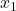to model the target variable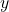. The degree 1 polynomial is the same as linear regression. For the degree 2 polynomial, we would add an additional feature which is the squared of. For the degree 3 polynomial, we would add two additional features – one which is the squared ofand the other which is the cubed of.The weights for the polynomial regression model can be obtained using the same algorithm as linear regression, i.e. the method of least squares using gradient descent. The best fit learned by each of the three polynomials is shown in Figure 4. We can see that the degree 3 polynomial fits the raw data better than degrees 2 and 1. We can interpret a polynomial regression model the same way as linear regression since the model is essentially a linear combination of the features including the higher degree features.

Polynomial regression however has some limitations. The complexity of the model increases as the number of features or the dimension of the feature space increases. It therefore has a tendency to overfit on the data. It is also hard to determine the degree for each feature in the polynomial especially in a higher dimensional feature space.
So, what model can be applied to overcome all these limitations and that’s interpretable? Enter, Generalized Additive Models (GAMs)! GAMs are models with medium to high predictive power and that are highly interpretable. Non-linear relationships are modeled using smoothing functions for each feature and by adding all of them. This is shown by the equation below.In the equation above, each feature has its own associated smoothing function that best models the relationship between that feature and the target. There are many types of smoothing functions that you can choose from but a widely used smoothing function is called regression splines as they are practical and computationally efficient. I will be focusing on regression splines throughout the book. Let’s now go deep into the world of GAMs using regression splines!
Regression Splines
Regression splines are represented as a weighted sum of linear or polynomial functions.These polynomial functions are also known as basis functions. This is shown mathematically below. In the equation,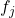is the function that models the relationship between the feature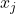and the target variable. This function is represented as a weighted sum of basis functions where the weight is represented as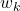and the basis function is represented as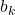. In the context of GAMs, the functionis called a smoothing function.Now, what is a basis function? A basis function is a family of transformations that can be used to capture a general shape or non-linear relationship. For regression splines, as the name suggests, splines are used as the basis function. A spline is a polynomial of degree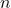with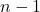continuous derivates. It will be much easier to understand splines using an illustration. Figure 3 shows splines of various degrees. The top left graph shows the simplest spline of degree 0, from which higher degree splines can be generated. As you can see from the top left graph, six splines have been placed on a grid. The idea is to split the distribution of the data into portions and fit a spline on each of those portions. So, in this illustration, the data has been split into six portions and we are modeling each portion as a degree 0 spline.
A degree 1 spline, shown in the top right graph, can be generated by convolving a degree 0 spline with itself. Convolution is a mathematical operation that takes in two functions and creates a third function that represents the correlation of the first function and a delayed copy of the second function. When we convolve a function with itself, we are essentially looking at the correlation of the function with a delayed copy of itself. There is a nice blog post by Christopher Olah on convolutions. This animation on Wikipedia will also give you a good intuitive understanding.By convolving a degree 0 spline with itself, we get a degree 1 spline which is triangular shaped, and this has a continuous 0th order derivative.
If we now convolve a degree 1 spline with itself, we will get a degree 2 spline shown in the bottom left graph. This degree 2 spline has a continuous 1st order derivative. Similarly, we can get a degree 3 spline by convolving a degree 2 spline and this has a continuous 2nd order derivative. In general, a degreespline has a continuousderivative. In the limit asapproaches infinity, we will obtain a spline that has the shape of a Gaussian distribution. In practice, degree 3 or cubic splines are used as it can capture most general shapes.
As mentioned earlier, in Figure 5 we have divided the distribution of data into six portions and have placed six splines on the grid. In the mathematical equation earlier, the number of portions or splines is represented as variable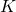. The idea behind regression splines is to learn the weights for each of the splines so that you can model the distribution of the data in each of the portions. The number of portions or splines in the grid,, is also called degrees of freedom. In general, if we place thesesplines on a grid, we will have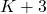points of division, also known as knots.

Let’s now zoom into cubic splines as shown in Figure 6. We can see that there are 6 splines or 6 degrees of freedom resulting in 9 points of division or knots.

Now to capture a general shape we will need to take a weighted sum of the splines. We will use cubic splines here. In Figure 7, we are using the same 6 splines overlaid to create 9 knots. For the graph on the left, I have set the same weights for all 6 splines. As you can imagine, if we take an equally weighted sum of all 6 splines, we will get a horizontal straight line. This is an illustration of a poor fit to the raw data. For the graph on the right however, I have taken an unequal weighted sum of the 6 splines generating a shape that perfectly fits the raw data. This shows the power of regression splines and GAMs. By increasing the number of splines or by dividing the data into more portions, we will have the ability to model more complex non-linear relationships. In GAMs based on regression splines, we individually model non-linear relationships of each feature with the target variable, and then add them all up to come up with the final prediction.

In Figure 7, the weights were determined using trial and error to best describe the raw data. But, how do you algorithmically determine the weights for a regression spline that best captures the relationship between the features and the target? Recall from earlier that a regression spline is a weighted sum of basis functions or splines. This is essentially a linear regression problem and you can learn the weights using the method of least squares and gradient descent. We would however need to specify the number of knots or degrees of freedom. We can treat this as a hyperparameter and determine it using a technique called cross-validation. Using cross-validation, we would remove a portion of the data and fit a regression spline with a certain number of pre-determined knots on the remaining data. This regression spline is then evaluated on the held-out set. The optimum number of knots is the one that results in the best performance on the held-out set.
In GAMs, you can easily overfit by increasing the number of splines or degrees of freedom. If the number of splines is high, the resulting smoothing function which is a weighted sum of the splines would be quite ‘wiggly,’ i.e. it would start to fit some of the noise in the data. How can we control this wiggliness or prevent overfitting? This can be done through a technique called regularization. In regularization, we would add a term to the least squares cost function that quantifies the wiggliness. The wiggliness of a smoothing function can be quantified by taking the integral of the squared of the 2nd order derivative of the function. By then using a hyperparameter (also called regularization parameter) represented by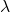, we can adjust the intensity of wiggliness. A high value forpenalizes wiggliness heavily. We can determinethe same way we determine other hyperparameters using cross-validation.

Summary of GAMs
A GAM is a powerful model where the target variable is represented as a sum of smoothing functions representing the relationship of each of the features and the target.The smoothing function can be used to capture any non-linear relationship. This is shown mathematically again below.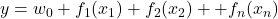It is a white-box model as we can easily see how each feature gets transformed to the output using the smoothing function. A common way of representing the smoothing function is using regression splines. A regression spline is represented as a simple weighted sum of basis functions. A basis function that is widely used for GAMs is the cubic spline. By increasing the number of splines or degrees of freedom, we can divide the distribution of data into small portions and model each portion piecewise. This way we can capture quite complex non-linear relationships. The learning algorithm essentially has to determine the weights for the regression spline. We can do this the same way as linear regression using the method of least squares and gradient descent. We can determine the number of splines using the cross-validation technique. As the number of splines increases, GAMs have a tendency to overfit on the data. We can safeguard against this by using the regularization technique. Using a regularization parameter , we can control the amount of wiggliness. Higherensures a smoother function. The parametercan also be determined using cross-validation.

GAMs can also be used to model interactions between variables. GA2M is a type of GAM that models pairwise interactions. It is shown mathematically below.With the help of Subject Matter Experts (SMEs), doctors in the Diagnostics+ example, you can determine what feature interactions need to be modeled. You could also look at the correlation between features to understand what features need to be modeled together.
In Python, there is a package called pyGAM that you can use to build and train GAMs. It is inspired by the GAM implementation in the popular mgcv package in R. You can install pyGAM in your Python environment using the pip package as follows.
pip install pygam
GAM for Diagnostics+ Diabetes
Let’s now go back to the Diagnostics+ example to train a GAM to predict diabetes progression using all 10 features. It is important to note that the sex of the patient is a categorical or discrete feature. It does not make sense to model this feature using a smoothing function. We can treat such categorical features in GAMs as factor terms. The GAM can be trained using the pyGAM package as follows. Please refer to the beginning of the article for the code that loads the diabetes dataset and that splits it into the train and test sets.
from pygam import LinearGAM #A
from pygam imports #B
from pygam import f #C # Load data using the code snippet in Section 2.2 gam = LinearGAM(s(0) + #D f(1) + #E s(2) + #F s(3) + #G s(4) + #H s(5) + #I s(6) + #J s(7) + #K s(8) + #L s(9), #M n_splines=35) #N gam.gridsearch(X_train, y_train) #O y_pred = gam.predict(X_test) #P mae = np.mean(np.abs(y_test - y_pred)) #Q #A Import the LinearGAM class from pygam that can be used to train a GAM for regression tasks
#B Import the smoothing term function to be used for numerical features
#C Import the factor term function to be used for categorical features
#D Cubic spline term for the Age feature
#E Factor term for the Sex feature which is categorical
#F Cubic splineterm for the BMI feature
#G Cubic spline term for the BP feature
#H Cubic spline term for the Total Cholesterol feature
#I Cubic spline term for the LDL feature
#J Cubic spline term for the HDL feature
#K Cubic spline term for the Thyroid feature
#L Cubic spline term for the Glaucoma feature
#M Cubic spline term for the Glucose feature
#N Maximum number of splines to be used for each feature
#O Using grid search to perform training and cross-validation to determine the number of splines, the regularization parameter lambda and the optimum weights for the regression splines for each feature
#P Use trained GAM model to predict on the test
#Q Evaluate the performance of the model on the test set using the MAE metric
Now for the moment of truth! How did the GAM perform? The MAE performance of the GAM is 41.4, a pretty good improvement when compared to the linear regression and decision tree models. A comparison of the performance of all 3 models is summarized in Table 1. I have also included the performance of a baseline model which Diagnostics+ and the doctors have been using where they looked at the median diabetes progression across all patients. All models are compared against the baseline to show how much of an improvement the models give to the doctors. It looks like GAM is the best model across all performance metrics!

MAE
RMSE
MAPE

Baseline
62.2
74.7
51.6

Linear Regression
42.8 (-19.4)
53.8 (-20.9)
37.5 (-14.1)

Decision Tree
48.6 (-13.6)
60.5 (-14.2)
44.4 (-7.2)

GAM
41.4 (-20.8)
52.2 (-22.5)
35.7 (-15.9)

Table 1: Performance comparison of Linear Regression, Decision Tree and GAM against a Baseline for Diagnostics+ AI
We have now seen the predictive power of GAMs. We could potentially get a further improvement in the performance by modeling feature interactions especially the cholesterol features with each other and with other features that are potentially highly correlated like BMI. As an exercise, I would highly encourage you to try modeling feature interactions using GAMs.
GAMs are white-box and can be easily interpreted. In the following section, we will see how GAMs can be interpreted.

GAMs can also be used to train a binary classifier by using the logistic link function where the response can be either 0 or 1. In the pyGAM package, you can make use of the logistic GAM for binary classification problems.
from pygam import LogisticGAM gam = LogisticGAM() gam.gridsearch(X_train, y_train)

Interpreting GAMs
Although each smoothing function is obtained as a linear combination of basis functions, the final smoothing function for each feature is non-linear and we therefore cannot interpret the weights the same way as linear regression. We however can easily visualize the effects of each feature on the target using partial dependence or partial effects plots. Partial dependence looks at the effect of each feature by marginalizing on the rest. It is highly interpretable as we can see the average effect of each feature value on the target variable. We can see if the target response to the feature is linear, non-linear, monotonic or non-monotonic.Figure 8 shows the effect of each of the patient metadata on the target variable. The 95% confidence interval around them have also been plotted.This will help us determine the sensitivity of the model to data points with low sample size.
Let’s now look at a couple of features in Figure 8 namely, BMI and BP. The effect of BMI on the target variable is shown by the bottom left graph. On the x-axis, we see the normalized values of BMI and on the y-axis, we see the effect that BMI has on the progression of diabetes for the patient. We see that as BMI increases, the effect on the progression of diabetes also increases. We see a similar trend for BP shown by the bottom right graph.We see that higher the BP, higher impact on the progression of diabetes. If we look at the 95% confidence internal lines (the dashed lines in Figure 8), we see a wider confidence internal around the lower and higher ends of BMI and BP. This is because there are fewer samples of patients at these range of values resulting in higher uncertainty in understanding the effects of these features at those ranges.

The code to generate Figure 8 is as follows.
grid_locs1 = [(0, 0), (0, 1), #A (1, 0), (1, 1)] #A
fig, ax = plt.subplots(2, 2, figsize=(10, 8)) #B
for i, feature in enumerate(feature_names[:4]): #C gl = grid_locs1[i] #D XX = gam.generate_X_grid(term=i) #E ax[gl, gl].plot(XX[:, i], gam.partial_dependence(term=i, X=XX)) #F ax[gl, gl].plot(XX[:, i], gam.partial_dependence(term=i, X=XX, width=.95), c='r', ls='--') #G ax[gl, gl].set_xlabel('%s' % feature) #H ax[gl, gl].set_ylabel('f ( %s )' % feature) #H #A Locations of the 4 graphs in the 2x2 matplotlib grid
#B Create 2x2 grid of matplotlib graphs
#C Iterate through the 4 patient metadata features
#D Get location of feature in the 2x2 grid
#E Generate the partial dependence of the feature values with the target marginalizing on the other features
#F Plot the partial dependence values as a solid line
#G Plot the 95% confidence interval around the partial dependence values as a dashed line
#H Add labels for the x and y axes
Figure 9 shows the effect of each of the 6 blood test measurements on the target. As an exercise, observe the effects that features like total cholesterol, LDL, HDL and glaucoma have on the progression of diabetes. What can you say about the impact of higher LDL values (or bad cholesterol) on the target variable? Why does higher total cholesterol have lower impact on the target variable? In order to answer these questions, let’s look at a few patient cases with very high cholesterol values. The code snippet below will help you zoom into those patients.
print(df_data[(df_data['Total Cholesterol'] &amp;gt; 0.15) &amp;amp;amp; (df_data['LDL'] &amp;gt; 0.19)])

If you execute the code above, you will see only 1 patient out of 442 that has a total cholesterol reading greater than 0.15 and an LDL reading greater than 0.19. The fasting glucose level for this patient one year out (the target variable) seems to be 84 which is in the normal range. This could explain why in Figure 9 we are seeing a very large negative effect for total cholesterol on the target variable for a range that is greater than 0.15. The negative effect of total cholesterol seems to be greater than the positive effect the bad LDL cholesterol seems to have on the target. The confidence interval seems much wider in these range of values. The model may have overfit on this one outlier patient record and so, we should not read too much into these effects. By observing these effects, we can identify cases or range of values where the model is sure of the prediction and cases where there is high uncertainty. For high uncertainty cases, we can go back to the diagnostics center to collect more patient data so that we have a representative sample.

Code to generate Figure 9 is as follows.
grid_locs2 = [(0, 0), (0, 1), #A (1, 0), (1, 1), #A (2, 0), (2, 1)] #A
fig2, ax2 = plt.subplots(3, 2, figsize=(12, 12)) #B
for i, feature in enumerate(feature_names[4:]): #C idx = i + 4 #D gl = grid_locs2[i] #D XX = gam.generate_X_grid(term=idx) #E ax2[gl, gl].plot(XX[:, idx], gam.partial_dependence(term=idx, X=XX)) #F ax2[gl, gl].plot(XX[:, idx], gam.partial_dependence(term=idx, X=XX, width=.95), c='r', ls='--') #G ax2[gl, gl].set_xlabel('%s' % feature) #H ax2[gl, gl].set_ylabel('f ( %s )' % feature) #H #A Locations of the 6 graphs in the 3x2 matplotlib grid
#B Create 3x2 grid of matplotlib graphs
#C Iterate through the 6 blood test measurement features
#D Get location of feature in the 3x2 grid
#E Generate the partial dependence of the feature values with the target marginalizing on the other features
#F Plot the partial dependence values as a solid line
#G Plot the 95% confidence interval around the partial dependence values as a dashed line
#H Add labels for the x and y axes
Through Figures 8 and 9, we can gain a much deeper understanding of the marginal effect of each of the feature values on the target. The partial dependence plots are useful to debug any issues with the model. By plotting the 95% confidence interval around the partial dependence values we can also see data points with low sample size. If feature values with low sample size has a dramatic effect on the target, then there could be an overfitting problem. We can also visualize the ‘wiggliness’ of the smoothing function to determine if the model has fit on the noise in the data. We can fix these overfitting problems by increasing the value of the regularization parameter. These partial dependence plots can also be shared with the SME, doctors in this case, for validation which will help gain their trust.
Limitations of GAMs
We have so far seen the advantages of GAMs in terms of predictive power and interpretability. GAMs have a tendency to overfit although this can be overcome with regularization. There are however some other limitations that you need to be aware of:

GAMs are sensitive to feature values outside of the range in the training set and tend to lose its predictive power when exposed to outlier values.
For mission critical tasks, GAMs may sometimes have limited predictive power, in which case you may need to consider more powerful black-box models.